JEE  >  NCERT Solutions Exercise 5.6: Continuity & Differentiability

# NCERT Solutions Exercise 5.6: Continuity & Differentiability - Mathematics (Maths) for JEE Main & Advanced

Continuity & Differentiability

Question 1:
If x and y are connected parametrically by the equation, without eliminating the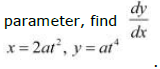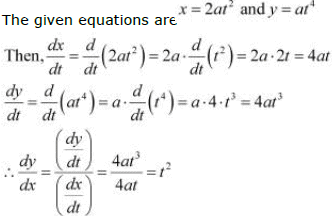Question 2: If x and y are connected parametrically by the equation, without eliminating the
parameter, find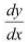.
x = a cos θ, y = b cos θ

The given equations are x = a cos θ and y = b cos θ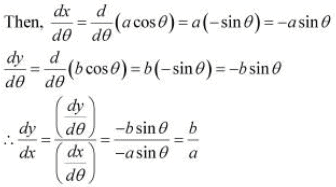Question 3: If x and y are connected parametrically by the equation, without eliminating the
parameter, find.
x = sin t, y = cos 2t

The given equations are x = sin t and y = cos 2t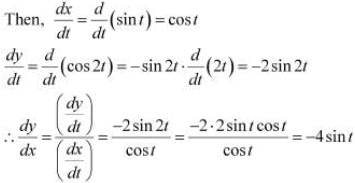Question 4:
If x and y are connected parametrically by the equation, without eliminating the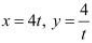.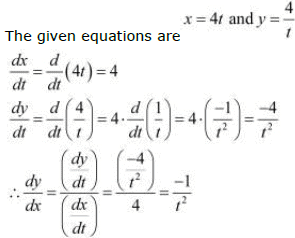Question 5: If x and y are connected parametrically by the equation, without eliminating the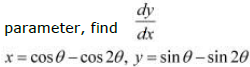The given equations are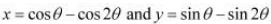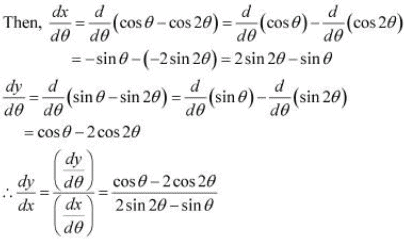Question 6: If x and y are connected parametrically by the equation, without eliminating the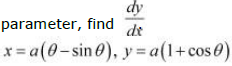The given equations are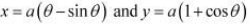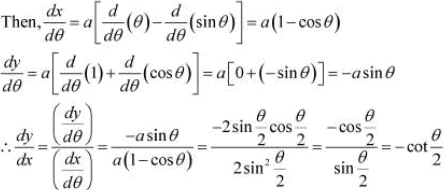Question 7: If x and y are connected parametrically by the equation, without eliminating the

parameter,  find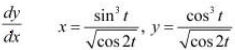.

The given equations are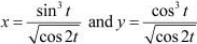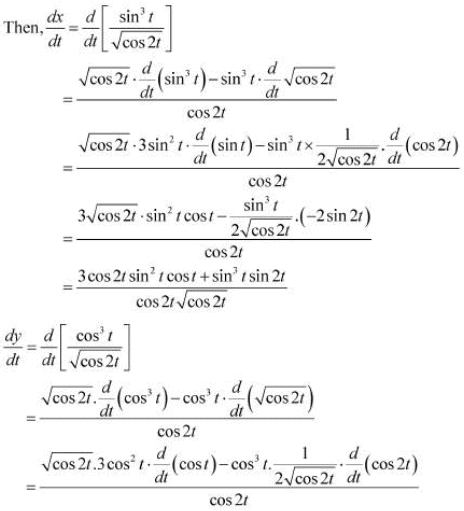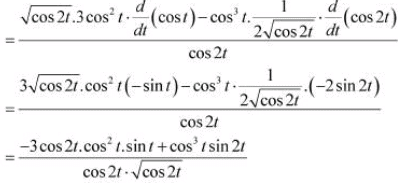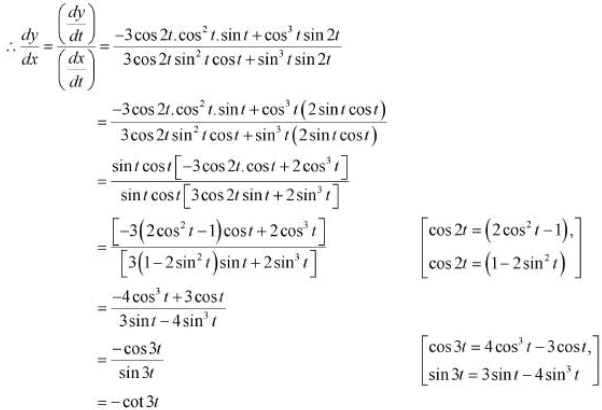Question 8: If x and y are connected parametrically by the equation, without eliminating the

parameter, find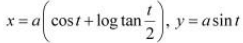The given equations are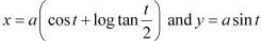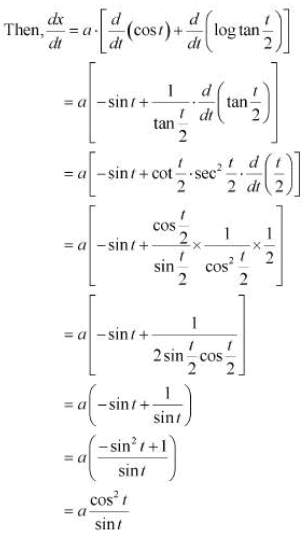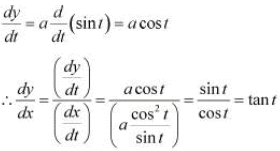Question 9: If x and y are connected parametrically by the equation, without eliminating the
parameter, find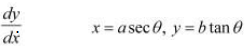.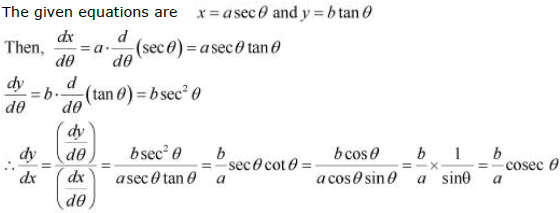Question 10: If x and y are connected parametrically by the equation, without eliminating the

parameter, find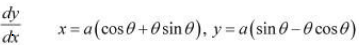.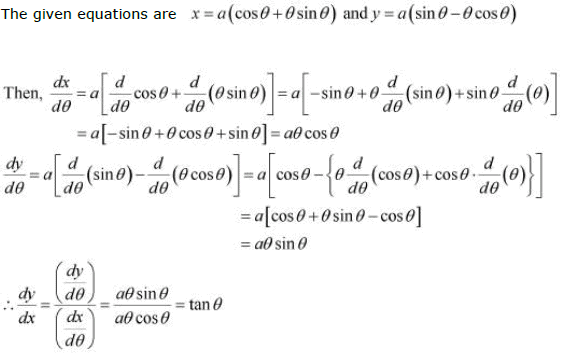Question 11:
If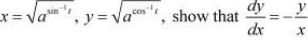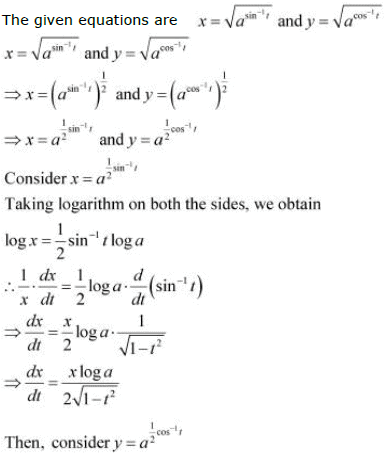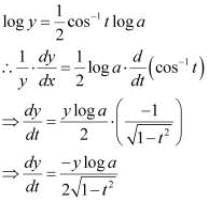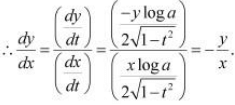Hence, proved.

The document NCERT Solutions Exercise 5.6: Continuity & Differentiability | Mathematics (Maths) for JEE Main & Advanced is a part of the JEE Course Mathematics (Maths) for JEE Main & Advanced.
All you need of JEE at this link: JEE

## FAQs on NCERT Solutions Exercise 5.6: Continuity & Differentiability - Mathematics (Maths) for JEE Main & Advanced

 1. What is continuity in calculus?Ans. Continuity is a fundamental concept in calculus that describes the behavior of a function. A function is said to be continuous at a point if it is defined at that point and the limit of the function as it approaches that point exists and is equal to the value of the function at that point. In simpler terms, a function is continuous if there are no breaks, jumps, or holes in its graph.
 2. How do you determine if a function is continuous or not?Ans. To determine if a function is continuous or not, you need to check three conditions: 1) The function must be defined at the point in question. 2) The limit of the function as it approaches that point must exist. 3) The value of the function at that point must be equal to the limit. If all three conditions are satisfied, the function is continuous at that point. If any of the conditions are not satisfied, the function is not continuous at that point.
 3. What is differentiability in calculus?Ans. Differentiability is another important concept in calculus. A function is said to be differentiable at a point if it is continuous at that point and has a derivative at that point. The derivative of a function represents its rate of change or slope at any given point.
 4. How do you determine if a function is differentiable or not?Ans. To determine if a function is differentiable or not, you need to check two conditions: 1) The function must be continuous at the point in question. 2) The derivative of the function must exist at that point. If both conditions are satisfied, the function is differentiable at that point. If any of the conditions are not satisfied, the function is not differentiable at that point.
 5. Can a function be continuous but not differentiable?Ans. Yes, it is possible for a function to be continuous but not differentiable. This can occur at points where the function has sharp corners, cusps, or vertical tangents. These are points where the derivative does not exist, even though the function itself is continuous. An example of such a function is the absolute value function, |x|. It is continuous but not differentiable at x = 0.

## Mathematics (Maths) for JEE Main & Advanced

129 videos|359 docs|306 tests

## Mathematics (Maths) for JEE Main & Advanced

129 videos|359 docs|306 tests
Signup to see your scores go up within 7 days! Learn & Practice with 1000+ FREE Notes, Videos & Tests.
10M+ students study on EduRev
Track your progress, build streaks, highlight & save important lessons and more!(Scan QR code)
Related Searches

,

,

,

,

,

,

,

,

,

,

,

,

,

,

,

,

,

,

,

,

,

;